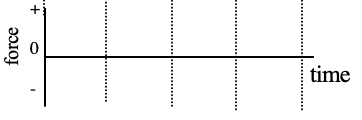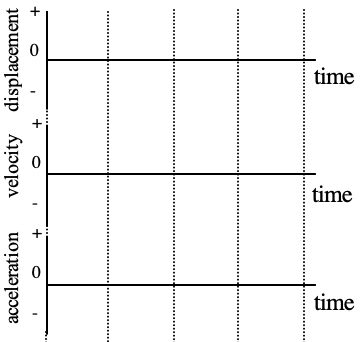Interactive Lecture Demonstration

Prediction Sheet—Simple Harmonic Motion

Directions:  Click here to download the Prediction Sheet.  Write your name at the top to record your presence and participation in these demonstrations.  Be sure to always make predictions before observing the results.  Your instructor may ask you to send this sheet in.

 Demonstration 1: We'll use a small cart that can oscillate between two springs. You can view the cart and springs by clicking here. The cart is interfaced with a computer that collects motion and force data as it oscillates. Its motion is started by pulling it to one side of its equilibrium position and releasing it. The displacement is zero whenever the cart is at its equilibrium position. You can download and view a video of the motion by clicking here. (Note that because of friction in the wheels, the amplitude of the motion decreases in time.) The force exerted on the cart by the springs is proportional to the displacement--the distance from the equilibrium point. Demonstration 1:  Watch the motion of the cart in the video and sketch on the axes on the right your predictions of the graphs of force vs. time, displacement vs. time, velocity vs. time, and acceleration vs. time. Be sure to carefully sketch the time relationship between the four quantities you are graphing. Only after you have made your predictions, click here to download a video showing the motion and the collected graphs. You can also download just the graphs by clicking here. Answer the following questions based on the graphs. A. What is the shape of the displacement vs. time graph? Do the other three graphs have a similar shape?   B. Find a positive maximum of the displacement. Are the maxima of velocity at the same times? If not what fraction of a period is the difference in time for the displacement and velocity maxima? Explain this difference in terms of the motion. When the cart is at its maximum displacement, what is the velocity?     C. Find a positive maximum of the displacement. Are the positive maxima of acceleration at the same times? If not what fraction of a period is the difference in time for the displacement and velocity maxima? Explain this difference in terms of the motion. When the cart is at its maximum displacement, what is the acceleration? When the cart is at its equilibrium position, what is the acceleration?         D. Compare the force-time graph to the acceleration-time graph.  How are they similar?  How different?  What is the relationship between force and acceleration? Is the force ever zero?  If so, at what point in the motion?Demonstration 2: Suppose that you wanted to model the motion (displacement) of the cart between two springs by writing down a mathematical expression for displacement vs. time.  What mathematical expression would you use?  What additional measurements would you need to make so that the expression would exactly represent the motion? Only after you have made your prediction, click here to see a possible mathematical expression representing the motion. For Demonstrations 3-5, you will be considering the motion of a mass (actually the same cart) suspended vertically from a spring. You can observe this motion by clicking here to download and view the video. Demonstration 3:  Suppose that increase the amplitude of the oscillation by pulling down the cart further below equilibrium before releasing it. Predict what effect this will have on the period and frequency of the motion. Only after you have made your prediction, click here to download a video and view the motion and a graph. You can also click here to view just the graph. Did increasing the amplitude of the oscillation affect the period and frequency? If yes, describe the change. Demonstration 4:  Suppose that increase the spring constant by suspending the cart with two springs next to each other. Predict what effect this will have on the period and frequency of the motion. Only after you have made your prediction, click here to download a video and view the motion and a graph. You can also click here to view just the graph. Did increasing the spring constant affect the period and frequency? If yes, describe the change. Demonstration 5: Suppose that increase the mass by hanging some weights from the bottom of the cart. Predict what effect this will have on the period and frequency of the motion. Only after you have made your prediction, click here to download a video and view the motion and a graph. You can also click here to view just the graph. Did increasing the mass affect the period and frequency? If yes, describe the change.